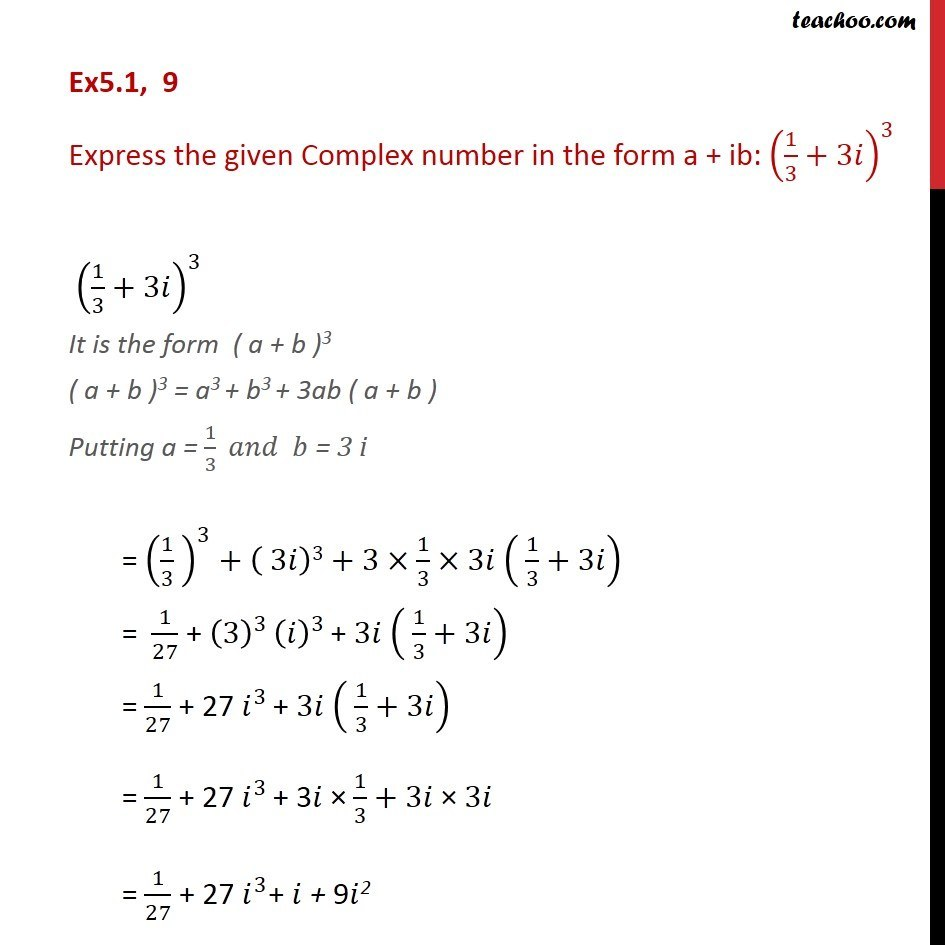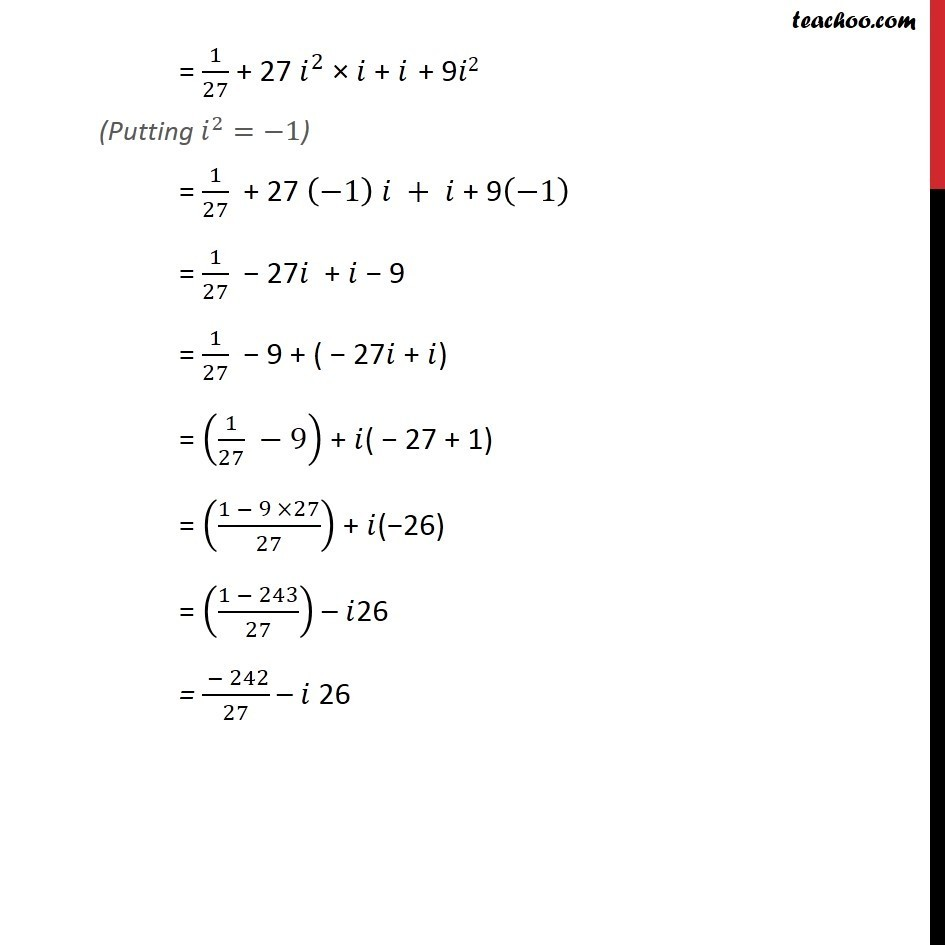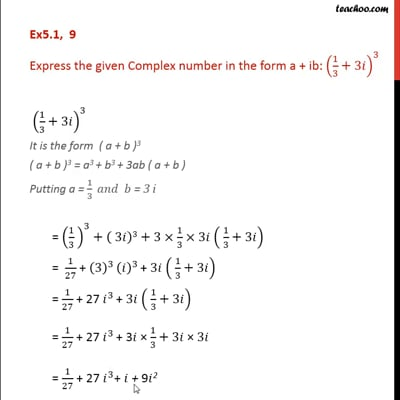Ex 5.1

Chapter 5 Class 11 Complex Numbers
Serial order wiseThis video is only available for Teachoo black users

Introducing your new favourite teacher - Teachoo Black, at only ₹83 per month

### Transcript

Ex5.1, 9 Express the given Complex number in the form a + ib: (1/3+3 )^3 (1/3+3 )^3 It is the form ( a + b )3 ( a + b )3 = a3 + b3 + 3ab ( a + b ) Putting a = 1/3 = 3 i = (1/3 )^3+( 3 )3+3 1/3 3 ( 1/3+3 ) = 1/27 + (3)^3 ( )^3 + 3 ( 1/3+3 ) = 1/27 + 27 ^3 + 3 ( 1/3+3 ) = 1/27 + 27 ^3 + 3 1/3+3 3 = 1/27 + 27 ^3+ + 9 2